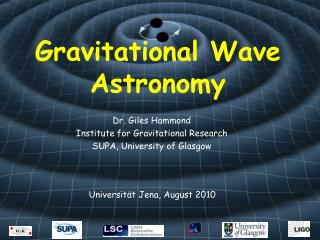DownloadDownload PresentationGravitational Wave Astronomy

# Gravitational Wave Astronomy

Télécharger la présentation## Gravitational Wave Astronomy

- - - - - - - - - - - - - - - - - - - - - - - - - - - E N D - - - - - - - - - - - - - - - - - - - - - - - - - - -
##### Presentation Transcript

1. Gravitational Wave Astronomy Dr. Giles Hammond Institute for Gravitational Research SUPA, University of Glasgow Universität Jena, August 2010

2. Gravitational Waves From our matrix all 3 terms are zero => A particle initially at rest will remain at rest. In the TT gauge we have a coordinate system which remains attached to individual particles Now consider two particles, one at (0,0,0) and the other at (,0,0) Proper distance between them is and this DOES change with time

3. Geodesic Deviation The geodesic deviation between two freely falling objects separated by a vector a is given by (not proved here) A fundamental result which states that curvature can be measured locally by watching the proper distance between particles If particles are at rest and separated by  along the x-axis then

4. Geodesic Deviation The components of the Riemann tensor can be calculated as So two particles separated by  along the x-axis will have a separation vector defined by

5. Example of Rxoxo Term

6. Geodesic Deviation The components of the Riemann tensor can be calculated as If the particles are separated by  along the y-axis it can be shown in a similar way that Previous result for x-axis

7. Geodesic Deviation For the hxx component The metric has sinusoidal solutions And this gives sinusoidal solutions for the particle separation from earlier slide

8. Geodesic Deviation Extending to a ring of test particles gives where there are 2 polarisations:

9. Leading Order Radiation Consider analogy with Electromagnetic radiation Waves are formed by the time-change in the position and distribution of the “charges” in the system (q or m) Monopole Radiation =>Time variation of total charge (zeroth moment) in the system Charge/Energy conservation rules this out for EW’s and GW’s

10. Leading Order Radiation Dipole Radiation => Time variation of the charge distribution (1st moment) Efficient production mechanism for EW’s Momentum conservation rules this out for GW’s (both linear + angular)

11. Leading Order Radiation Quadrupole Radiation => Time variation of the charge distribution (2nd moment) No conservation rules left => leading order radiation term for GW’s 2nd moment depends on the moment of inertia tensor (Lij)

12. Estimate of Strain Amplitude Consider two stars, mass M, radial separation r The quadrupole moment is R R

13. Estimate of Strain Amplitude The magnitude of the metric stretch in the xx direction is or, using Kepler’s 3rd law, which gives modulated at 2